SLOS954A July   2018  – December 2018

PRODUCTION DATA.

1. Features
2. Applications
3. Description
4. Revision History
5. Device Comparison Table
6. Pin Configuration and Functions
7. Specifications
8. Detailed Description
1. 8.1 Overview
2. 8.2 Functional Block Diagram
3. 8.3 Feature Description
4. 8.4 Device Functional Modes
9. Application and Implementation
1. 9.1 Application Information
2. 9.2 Typical Applications
1. 9.2.1 High-Side, High-Drive, Solenoid Current-Sense Application
2. 9.2.2 Speaker Enhancements and Diagnostics Using Current Sense Amplifier
3. 9.2.3 Current Sensing for Remote I/Os in PLC
10. 10Power Supply Recommendations
11. 11Layout
12. 12Device and Documentation Support
13. 13Mechanical, Packaging, and Orderable Information

• PW|20

### 7.6 Typical Characteristics

at TA = 25°C, VS = 5 V, VIN+ = 12 V, and VREF = VS / 2 (unless otherwise noted)All gains
Figure 1. Input Offset Voltage Production Distribution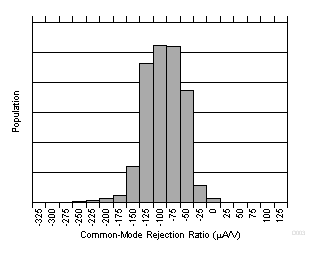All gains
Figure 3. Common-Mode Rejection Production Distribution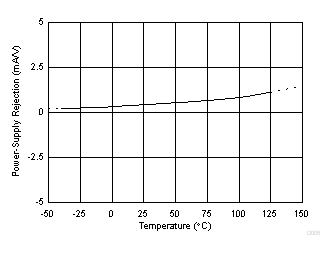Figure 5. Power-Supply Rejection Ratio vs Temperature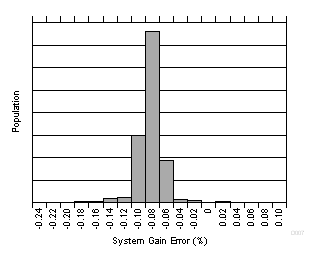Figure 7. Gain Error Production Distribution (INA253A2)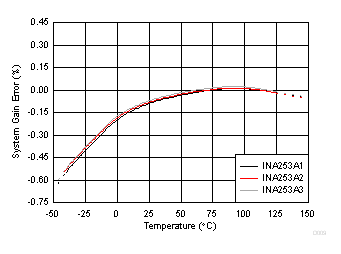Figure 9. System Gain Error vs Temperature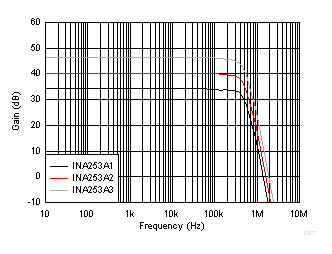VCM = 0 V, VDIFF = 10-mVPP sine
Figure 11. Amplifier Gain Error vs Frequency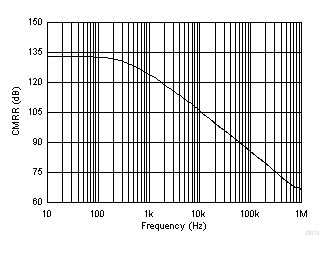Figure 13. Common-Mode Rejection Ratio vs Frequency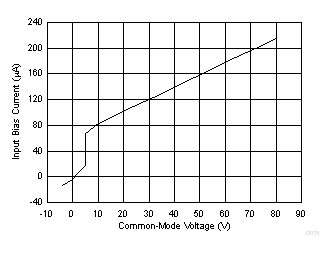VS = 5 V
Figure 15. Input Bias Current vs Common-Mode Voltage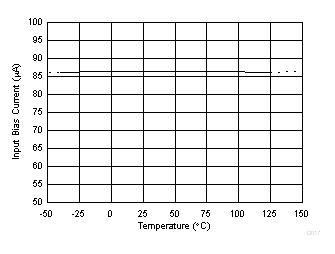Figure 17. Input Bias Current vs Temperature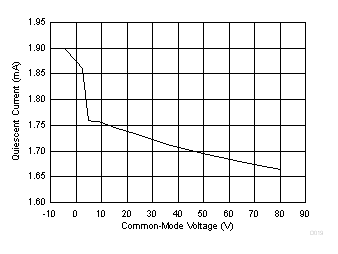Figure 19. Quiescent Current vs Common-mode Voltage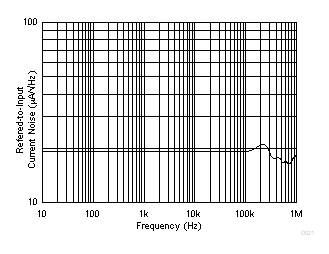Figure 21. INA253A2 Input-Referred Voltage Noise vs Frequency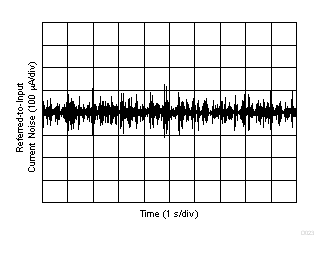Figure 23. 0.1-Hz to 10-Hz Voltage Noise (Referred-to-Input)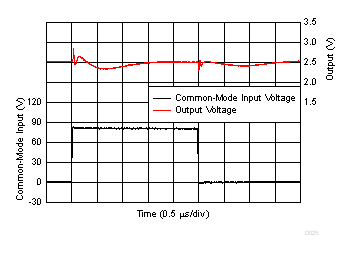VREF1 = VREF2 = 0 V
Figure 25. Common-Mode Transient Response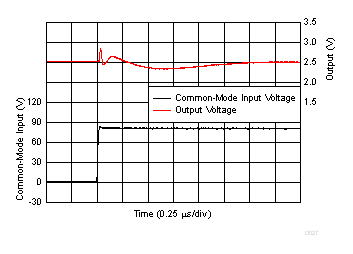Rising Edge
Figure 27. Common-Mode Voltage Transient Response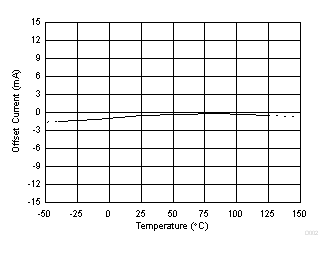Figure 2. Input Offset Current vs TemperatureFigure 4. Common-Mode Rejection Ratio vs Temperature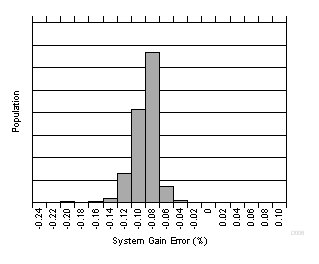Figure 6. Gain Error Production Distribution (INA253A1)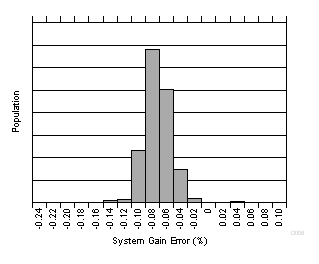Figure 8. Gain Error Production Distribution (INA253A3)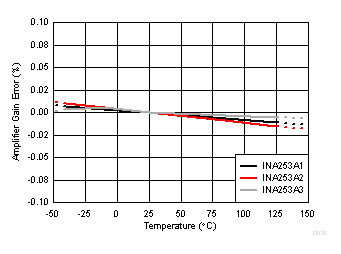Figure 10. Amplifier Gain Error vs Temperature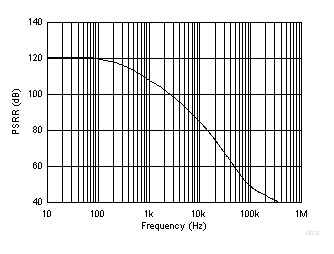Figure 12. Power-Supply Rejection Ratio vs Frequency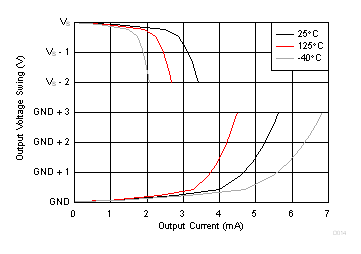VS = 5 V
Figure 14. Output Voltage Swing vs Output CurrentVS = 0 V
Figure 16. Input Bias Current vs Common-Mode Voltage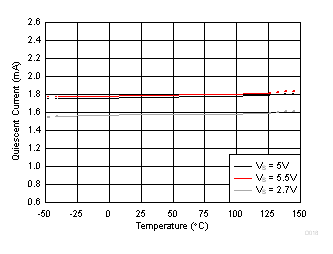Figure 18. Quiescent Current vs TemperatureFigure 20. INA253A1 Input-Referred Voltage Noise vs Frequency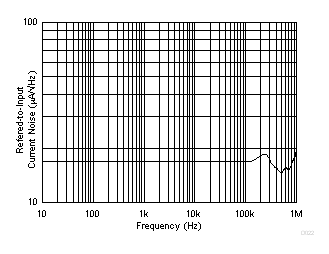Figure 22. INA253A3 Input-Referred Voltage Noise vs Frequency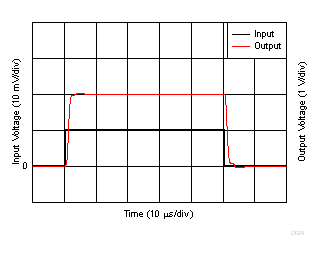VREF1 = VREF2 = 0 V, 10-mVPP input step
Figure 24. Amplifier Step Response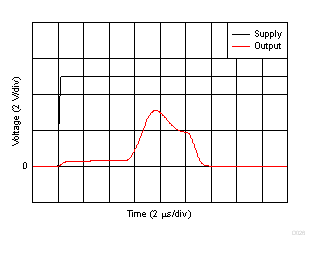Figure 26. Start-Up ResponseFalling Edge
Figure 28. Common-Mode Voltage Transient Response